### Digital Logic Circuits–Multiplexer and De-multiplexer

Multiplexer

A multiplexer (MUX) is a digital switch which connects data from one of n sources to the output. A number of select inputs determine which data source is connected to the output. The block diagram of MUX with n data sources of b bits wide and s bits wide select line is shown in below figure.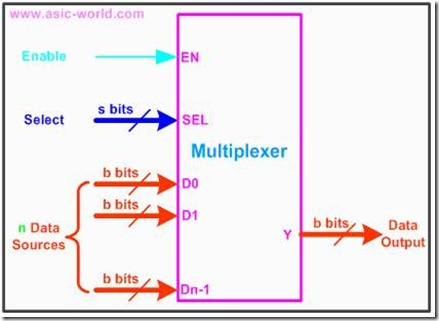MUX acts like a digitally controlled multi-position switch where the binary code applied to the select inputs controls the input source that will be switched on to the output as shown in the figure below. At any given point of time only one input gets selected and is connected to output, based on the select input signal.

The operation of a multiplexer can be better explained using a mechanical switch as shown in the figure below. This rotary switch can touch any of the inputs, which is connected to the output. As you can see at any given point of time only one input gets transferred to output.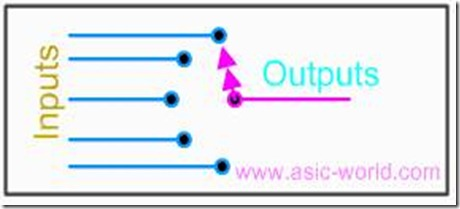2x1 MUX

A 2 to 1 line multiplexer is shown in figure below, each 2 input lines A to B is applied to one input of an AND gate. Selection lines S are decoded to select a particular AND gate. The truth table for the 2:1 mux is given in the table below.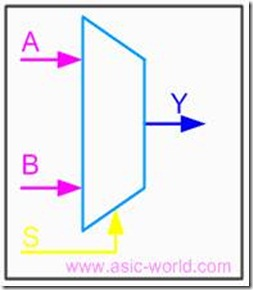Design of a 2:1 Mux

To derive the gate level implementation of 2:1 mux we need to have truth table as s hown in figure. And once we have the truth table, we can draw the K-map as shown in figure for all the cases when Y is equal to '1'.

Combining the two 1' as shown in figure, we can drive the output y as shown below

Y = A.S’ + B.S

Truth Table

 B A S Y 0 0 0 0 0 0 1 0 0 1 0 1 0 1 1 0 1 0 0 0 1 0 1 1 1 0 1 1 1 1 1

Kmap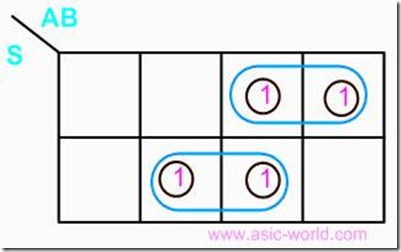Circuit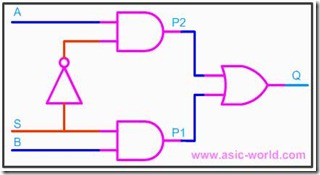MSI MUX

74150: 16-to-1

74153: Dual 4-to-1

74151: 8-to-1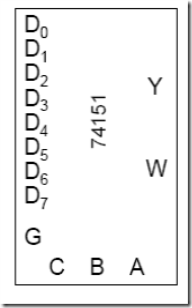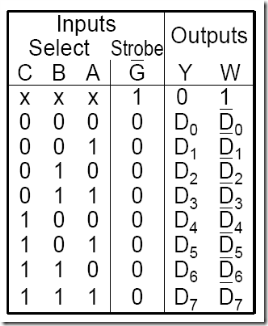16-to-1 MUX

Use two 74151

D = 0 enables top MUX

D = 1 enables bottom MUX

W = Y’

= (Y1+Y2)’

= (W1’+W2’)’

= W1W2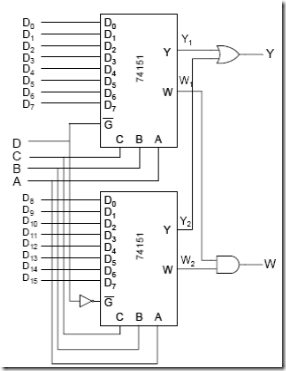Larger Multiplexers

Larger multiplexers can be constructed from smaller ones. An 8-to-1 multiplexer can be constructed from smaller multiplexers as shown below.

8-to-1 multiplexer from Smaller MUX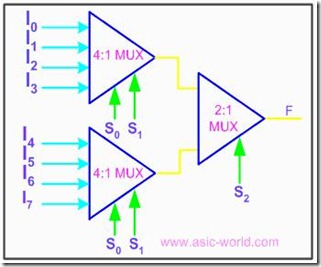16-to-1 multiplexer from 4:1 mux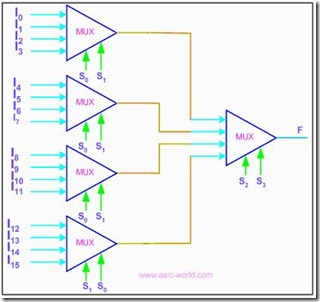It is 2-to-1 MUX with 4 bits for each input

There is 1 output of 4 bits

There is 1 select signal

When 1 input is selected, the whole group of 4 bits goes to the output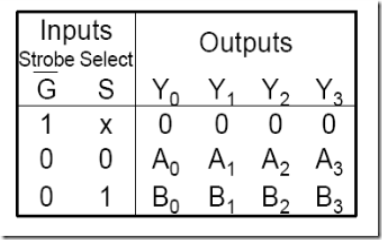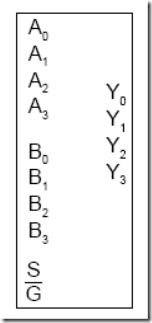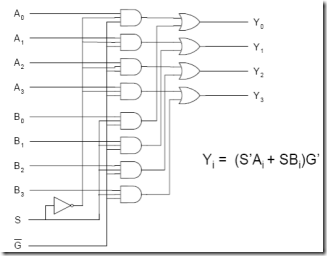Implementing Functions Multiplexers

Any n-variable logic function can be implemented using a smaller 2n-1-to-1 multiplexer and a single inverter (e.g 4-to-1 mux to implement 3 variable functions) as follows.

Express function in canonical sum-of-minterms form. Choose n-1 variables as inputs to mux select lines. Construct the truth table for the function, but grouping inputs by selection line values (i.e select lines as most significant inputs).

Determine multiplexer input line i values by comparing the remaining input variable and the function F for the corresponding selection lines value i.

We have four possible mux input line i values:

· Connect to 0 if the function is 0 for both values of remaining variable.

· Connect to 1 if the function is 1 for both values of remaining variable.

· Connect to remaining variable if function is equal to the remaining variable.

· Connect to the inverted remaining variable if the function is equal to the remaining variable inverted

3-variable Function Using 8-to-1 mux

Implement the function F(X,Y,Z) = S(1,3,5,6) using an 8-to-1 mux. Connect the input variables X, Y, Z to mux select lines. Mux data input lines 1, 3, 5, 6 that correspond to the function minterms are connected to 1. The remaining mux data input lines 0, 2, 4, 7 are connected to 0.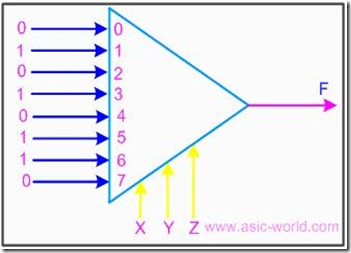3-variable Function Using 4-to-1 mux

Implement the function F(X,Y,Z) = S(0,1,3,6) using a single 4-to-1 mux and an inverter. We choose the two most significant inputs X, Y as mux select lines.

Truth Table

 Select i X Y Z F Mux Input i 0 0 0 0 1 1 0 0 0 1 1 1 1 0 1 0 0 Z 1 0 1 1 1 Z 2 1 0 0 0 0 2 1 0 1 0 0 3 1 1 0 1 Z' 3 1 1 1 0 Z'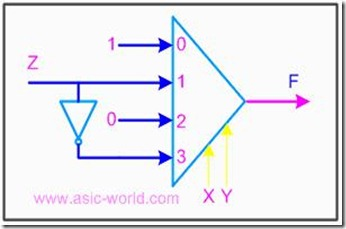We determine multiplexer input line i values by comparing the remaining input variable Z and the function F for the corresponding selection lines value i

· when XY=00 the function F is 1 (for both Z=0, Z=1) thus mux input0 = 1

· when XY=01 the function F is Z thus mux input1 = Z

· when XY=10 the function F is 0 (for both Z=0, Z=1) thus mux input2 = 0

· when XY=11 the function F is Z' thus mux input3 = Z'

Example for logic function implementation using MUX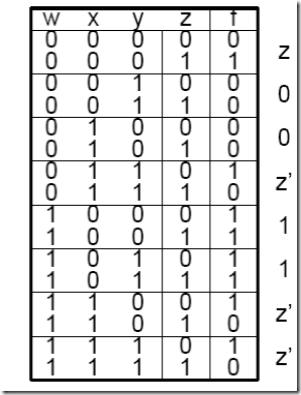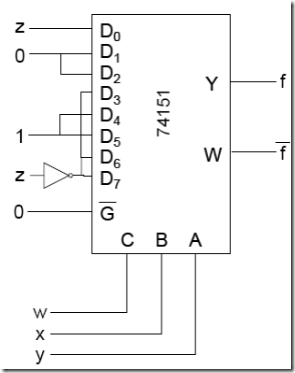De-multiplexers

They are digital switches which connect data from one input source to one of n outputs.Usually implemented by using n-to-2n binary decoders where the decoder enable line is used for data input of the de-multiplexer.The figure below shows a de-multiplexer block diagram which has got s-bits-wide select input, one b-bits-wide data input and n b-bits-wide outputs.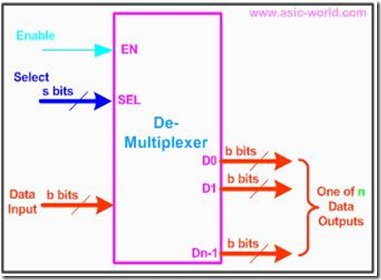The operation of a de-multiplexer can be better explained using a mechanical switch as shown in the figure below. This rotary switch can touch any of the outputs, which is connected to the input. As you can see at any given point of time only one output gets connected to input.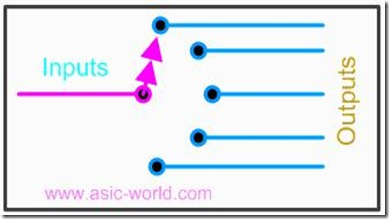1-to-4 De-multiplexer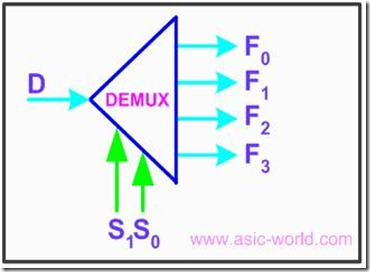Truth Table

 S1 S0 F0 F1 F2 F3 0 0 D 0 0 0 0 1 0 D 0 0 1 0 0 0 D 0 1 1 0 0 0 D